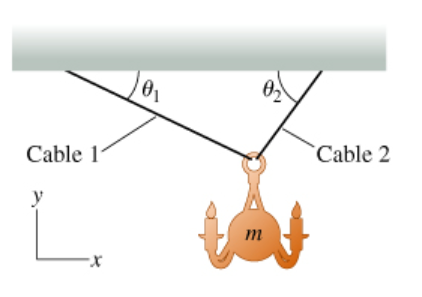# Problem: Hanging Chandelier (Figure 1 A chandelier with mass m is attached to the ceiling of a large concert hall by two cables. Because the ceiling is covered with intricate architectural decorations (not indicated in the figure, which uses a humbler depiction), the workers who hung the chandelier couldn't attach the cables to the ceiling directly above the chandelier Instead, they attached the cables to the ceiling near the walls. Cable 1 has tension Ti and makes an angle θ1 with the ceiling. Cable 2 has tension T2 and makes an angle θ2 with the ceiling.Find an expression for T1, the tension in cable 1, that does not depend on T2.Express your answer in terms of some or all of the variables m, θ1, and θ2, as well as the magnitude of the acceleration due to gravity g. You must use parentheses around θ1 and θ2, when they are used as arguments to any trigonometric functions in your answer.

###### FREE Expert Solution

The forces are balanced in the vertical direction.

Therefore:

$\overline{){\mathbf{\Sigma }}{\mathbf{F}}{\mathbf{=}}{\mathbf{0}}}$

${\mathbit{T}}_{\mathbf{1}}\mathbit{s}\mathbit{i}\mathbit{n}{\mathbit{\theta }}_{\mathbf{1}}\mathbf{+}{\mathbit{T}}_{\mathbf{2}}\mathbit{s}\mathbit{i}\mathbit{n}{\mathbit{\theta }}_{\mathbf{2}}\mathbf{=}\mathbit{m}\mathbit{g}$

The forces are also balanced in the horizontal direction.

Fnet = 0

${\mathbit{T}}_{\mathbf{1}}\mathbit{c}\mathbit{o}\mathbit{s}{\mathbit{\theta }}_{\mathbf{1}}\mathbf{=}{\mathbit{T}}_{\mathbf{2}}\mathbit{s}\mathbit{i}\mathbit{n}{\mathbit{\theta }}_{\mathbf{2}}$

${\mathbit{T}}_{\mathbf{2}}\mathbf{=}{\mathbit{T}}_{\mathbf{1}}\mathbf{\left(}\mathbit{c}\mathbit{o}\mathbit{s}{\mathbit{\theta }}_{\mathbf{1}}\mathbf{\right)}\mathbf{/}\mathbf{\left(}\mathbit{c}\mathbit{o}\mathbit{s}{\mathbit{\theta }}_{\mathbf{2}}\mathbf{\right)}$

90% (379 ratings)###### Problem Details

Hanging Chandelier (Figure 1 A chandelier with mass m is attached to the ceiling of a large concert hall by two cables. Because the ceiling is covered with intricate architectural decorations (not indicated in the figure, which uses a humbler depiction), the workers who hung the chandelier couldn't attach the cables to the ceiling directly above the chandelier Instead, they attached the cables to the ceiling near the walls. Cable 1 has tension Ti and makes an angle θ1 with the ceiling. Cable 2 has tension T2 and makes an angle θ2 with the ceiling.Find an expression for T1, the tension in cable 1, that does not depend on T2.

Express your answer in terms of some or all of the variables m, θ1, and θ2, as well as the magnitude of the acceleration due to gravity g. You must use parentheses around θ1 and θ2, when they are used as arguments to any trigonometric functions in your answer.